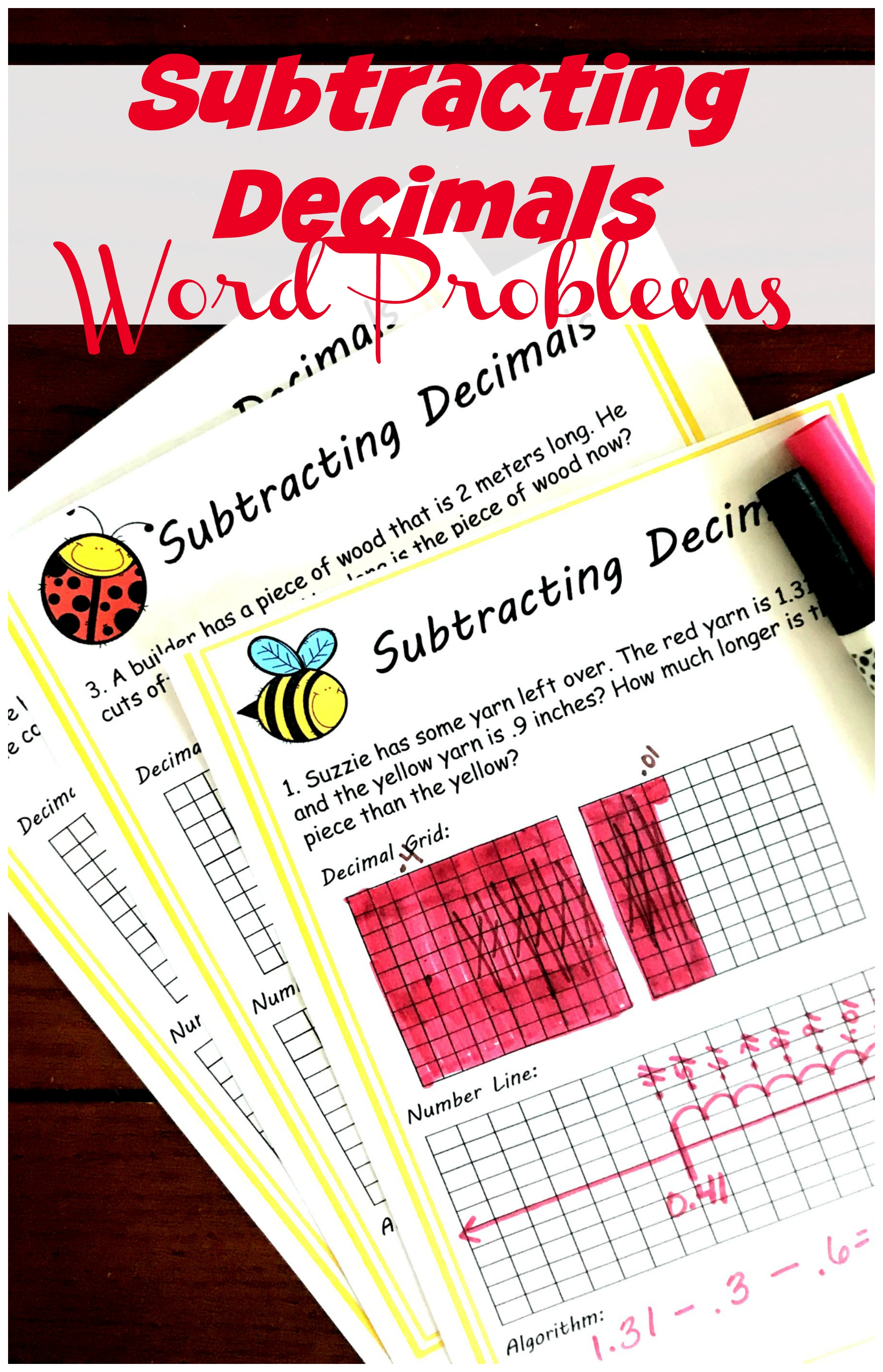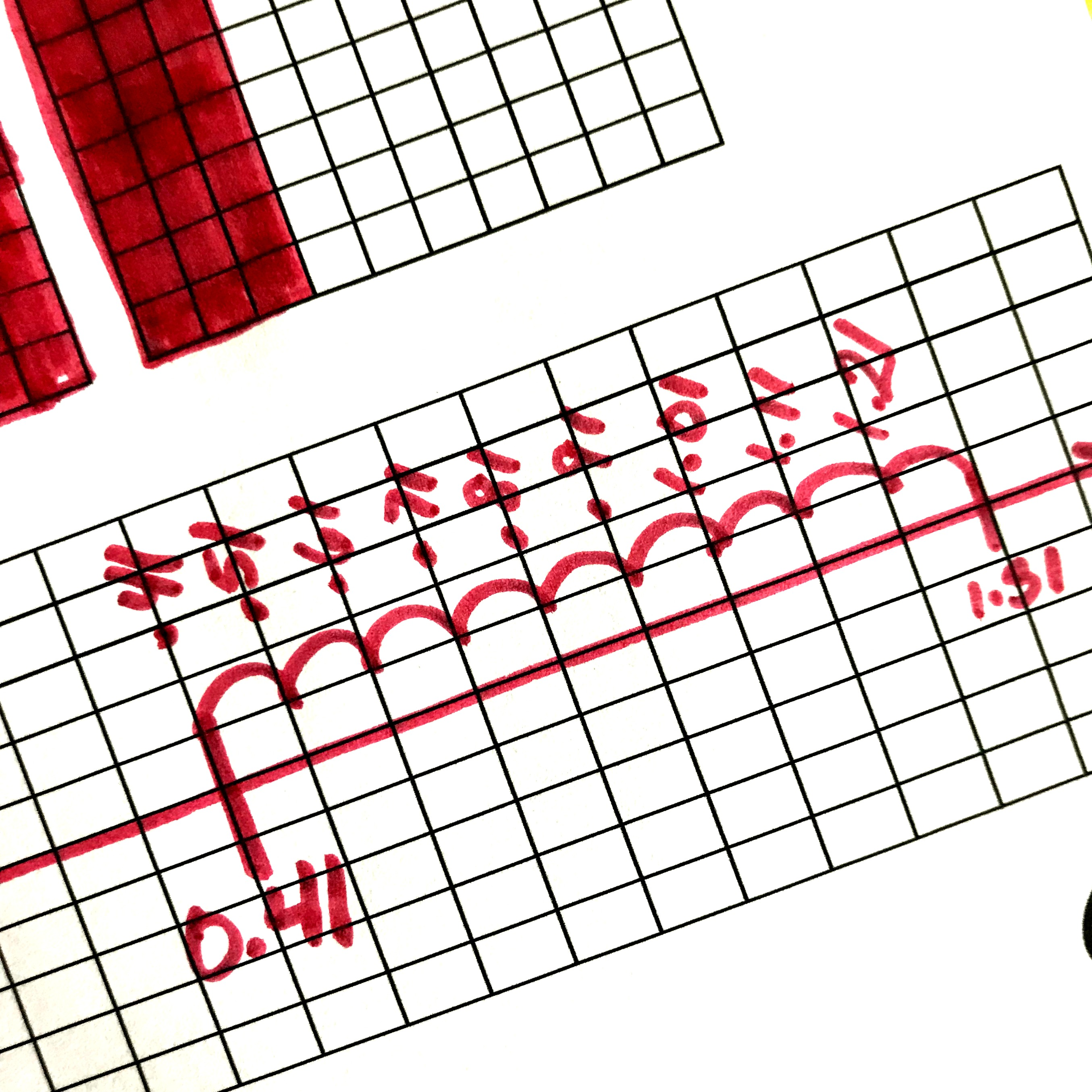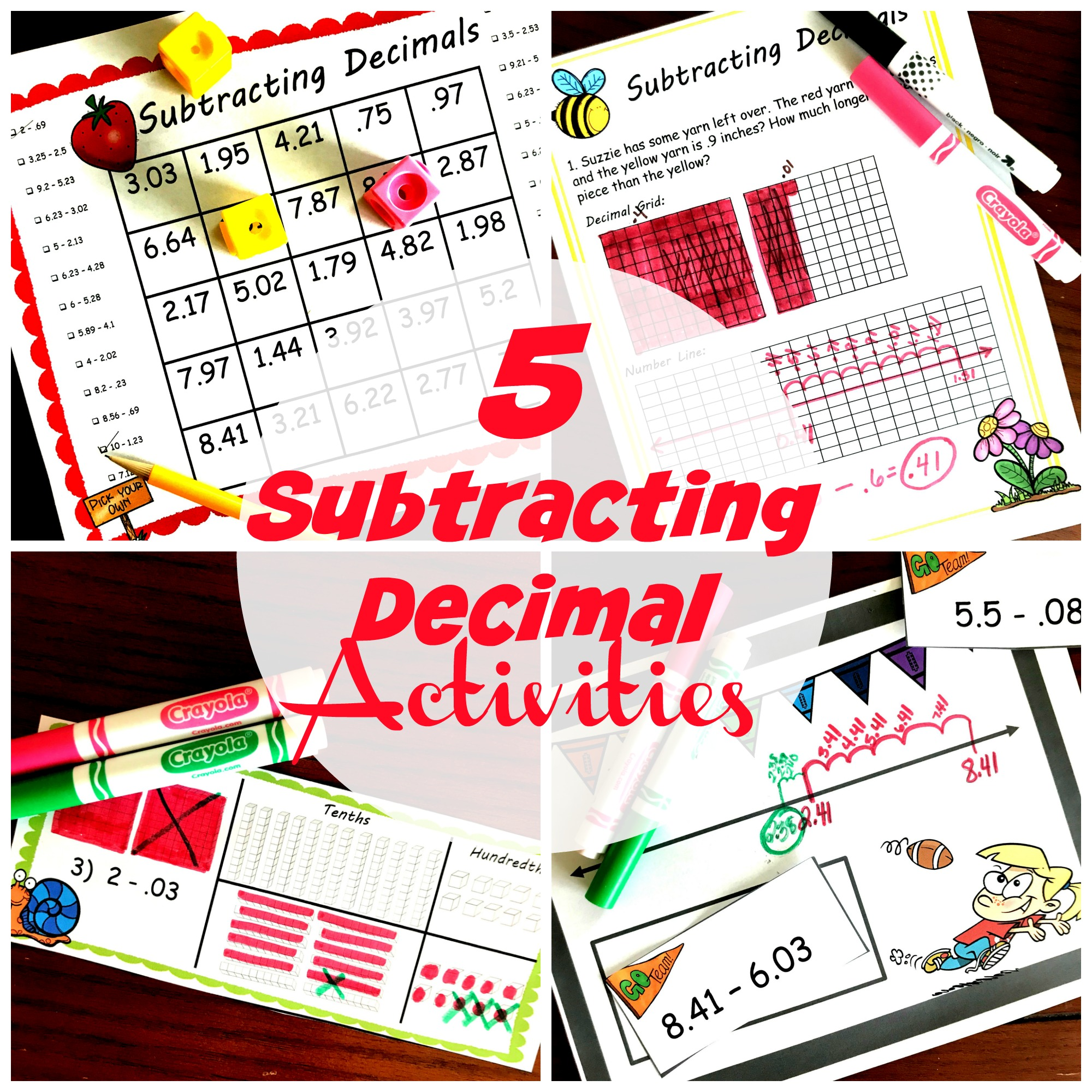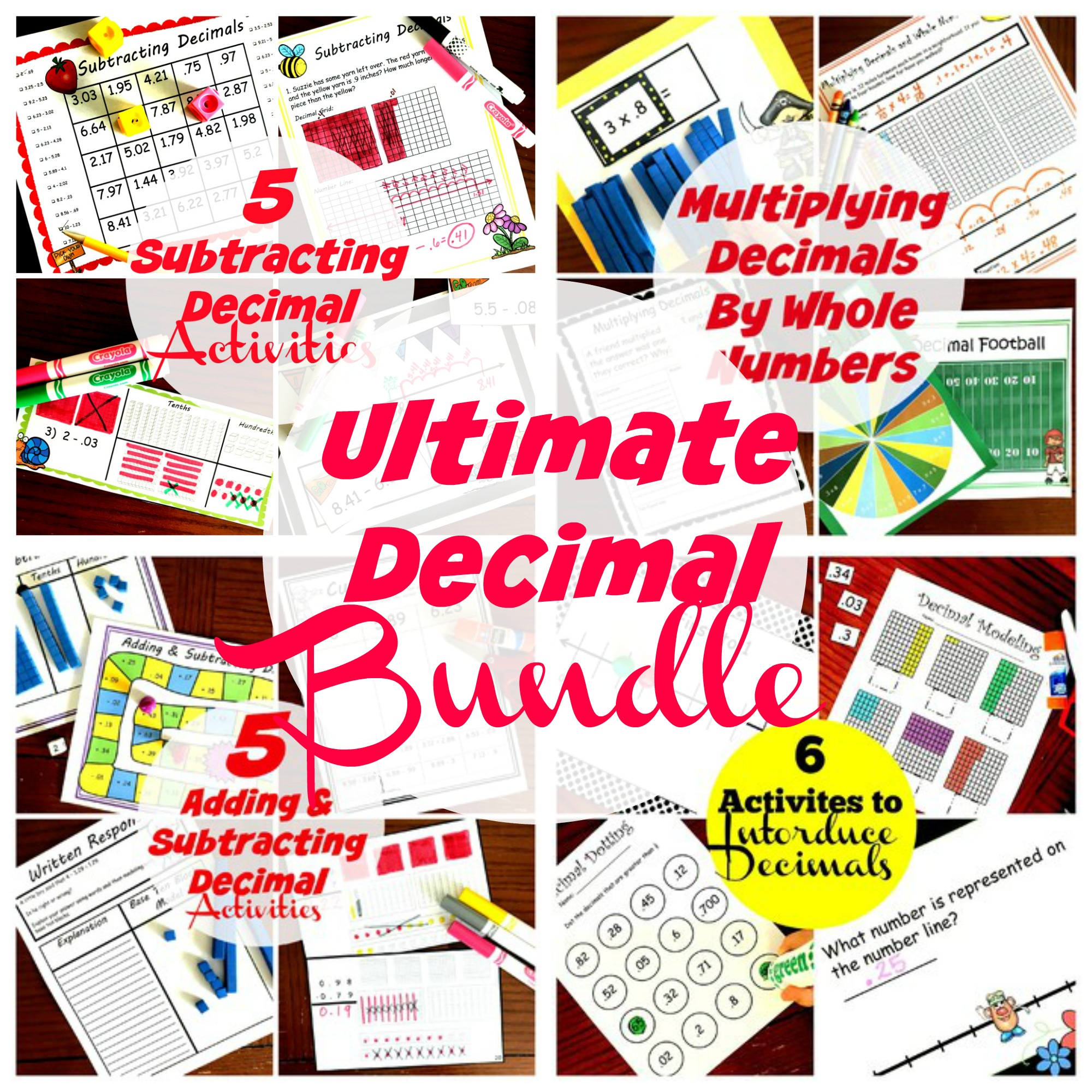Home » Math » FREE Hands-On Subtracting Decimals Word Problems

FREE Hands-On Subtracting Decimals Word Problems

Your students will have fun solving subtraction decimal word problems. They are hands-on and will help our visual learners be successful.It is time for some new word problems that focus on subtracting decimals. These interactive, hands-on word problems are a fun way to visualize the process.

SO grab some crayons and pencils and let’s get solving these subtracting decimal word problems.

Subtracting Decimal Word Problems

There are three different ways for our children to solve these subtraction problems. Each way builds number sense and provides strategies that could help your students be able to solve these types of problems mentally.

Decimal Grids:

When using the decimal grids, you begin by coloring in the minuend (the first number in the subtraction expression). In this example, we are coloring in 1.31.

One stands for one whole.

The three stands for 3 tenths so we color in 3 out of the ten rows.

The last one stands for 1 hundredth. So we color in one out of a hundred squares.

(If this is hard for your students, it may be a good idea to start here.)Now it is time to subtract. The subtrahend (second number in the subtraction expression) tells us how many we need to cross off.

In this example, it is .9 or nine-tenths.

So we are going to cross off nine columns.Now, all we have to do is count up what is left.

There are four tenths and one hundredth. So the answer is 0.41

Number Lines:

Now we move onto number lines. There are two ways we can do this.

One way is to count backward.

We start by writing the minuend on the number line. Then we count backward.

With this example, we only have to subtract nine-tenths. So we count back by tenths.

1.21, 1.11, 1.01, 0.91, 0.81, 0.71, 0.61, 0.51, 0.41For another way to subtract on a number line, check out this video.

Equations:

Finally, we get to equations. This is a way to break numbers apart or decompose the original expression.

I’m starting with my minuend (1.31) and trying to figure out an easy way to subtract from there. Well, I know that if I subtract .3 that puts me at 1.01. Now I know that I needed to subtract another .6, and this is easy.

10 – 6 = 4…so 1.01 – .6 = .41There is so much thinking involved while solving these word problems, and tons of number sense is being developed. It may take a little more time, but the understanding that comes from it will be beneficial.

You’ve Got This,

Rachel

Want more subtraction work? Check out this subtraction bundle.Or get all my decimal activities in one easy download.